##1. Harmonic bundle

We say that a bundle (or pencil) of four lines (CA, CB, CG, CH) passing through a common point C is a [harmonic bundle] of lines, if there is some line [AB] intersecting all four lines of the bundle at points A, B, G, H, such that G, H are harmonically conjugate to A, B, i.e. the oriented ratios are equal: GA/GB = -HA/HB (see Harmonic.html ).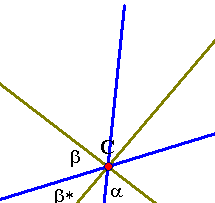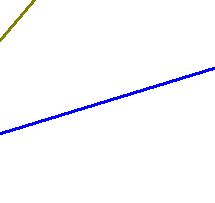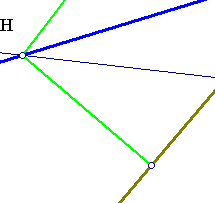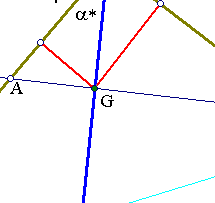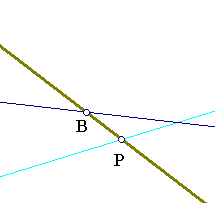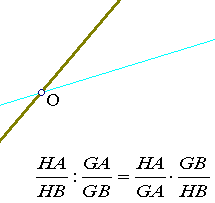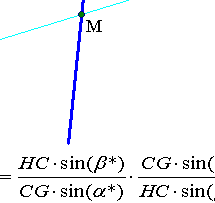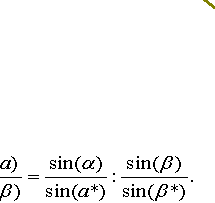The previous calculation shows that the cross ratio (A,B,G,H) is a ratio of the sines of the angles between the lines (see also ProjectiveLine.html ). Hence it is the same for every line intersecting these four lines. Thus, if a line crossing a bundle defines four harmonically conjugate points (i.e. (A,B,G,H)=-1), the same must be true for every other line intersecting this bundle.
This simple fact has many implications, some of them discussed below.

##2. Harmonic bundle with two orthogonals

If the bundle is harmonic and the lines CH, CG are orthogonal, then they are the bisectors of the two other lines.
In fact, in this case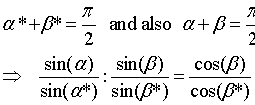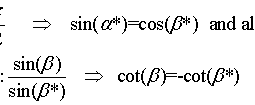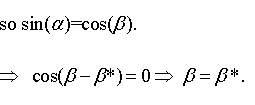##3. Harmonic bundle and parallels

If the bundle is harmonic and we draw a line L parallel to CH, then CG passes through the middle M of the segment OP on L, determined by the other two lines. The reason is that the middle M is the harmonic conjugate to A, B of the point at infinity of line L, for which the ratio HA/HB tends to the limiting position 1, hence MO/MP = -1. The inverse is also true: If a bundle of four lines through a point (CH, CA, CG, CB) has the property that a segment (DB) parallel to CH is divided by the other three lines of the bundle in two equal parts (DE=EB), then the bundle is harmonic. For this it suffices to calculate the ratios in the following figure, G being the centroid of triangle BCD.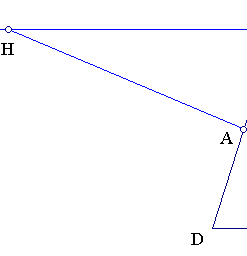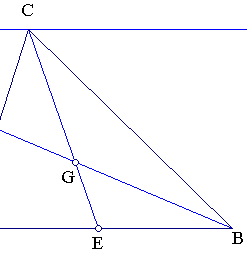A well known variation of this rule is that two adjacent sides of the parallelogram and its diagonals (drawn parallel from the common vertex) build a harmonic bundle.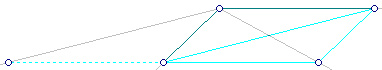##4. Application to triangle-altitudes

Consider a point P on the altitude AD of triangle ABC and assume that BE, CF pass through P. Then AD is the bisector of angle EDF, see the figure below.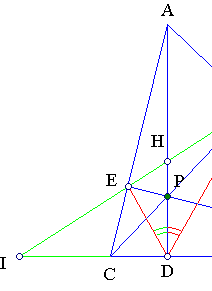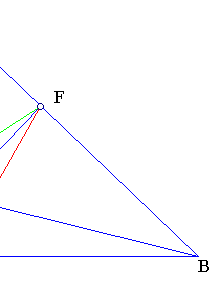To prove this it suffices to apply (2) to the bundle of lines at D: (DE, DF, DH, DI), which form a harmonic bundle of lines (see Harmonic.html ).

There is another aspect of the invariance of the cross ratio of four lines intercepted by a fifth. This is the aspect of line-perspectivity, defined by a pencil of lines C* (C* denoting all lines through C) on two other lines {e1,e2} intersecting the lines of this bundle. This is discussed in ProjectiveLine.html and RectHypeRelation.html .

##5. Application to orthogonal chords

Let {AB,CD} be two orthogonal chords of a circle intersecting at point E. Consider the intersection point F of {AD,BC} and draw an orthogonal to line OE (O the center of the circle) at E intersecting {BC,AD} correspondingly at {K,L}. Then KE = EL.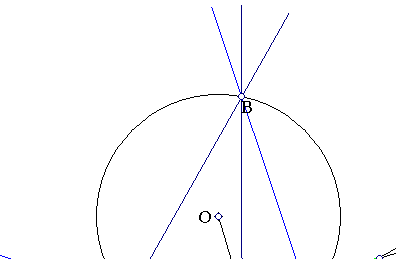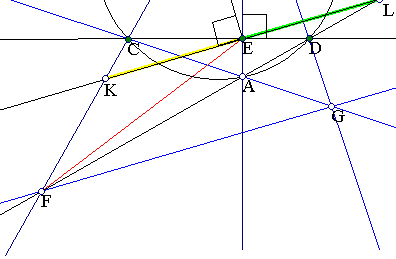The proof follows immediately by completing the figure to the complete quadrilateral defined by the four points {A,B,C,D}. Line FG is the polar of point E (see Polar_Construction.html ) and as such it is orthogonal to OE as is also KL. Since the bundle of lines F(A,B,E,G) is harmonic and KL is parallel to line FG the result of section 3 applies.
For another application in this context see Harmonic_Bundle2.html .

Autopolar.html
Butterfly.html
CrossRatio0.html
CrossRatioLines.html
Complex_Cross_Ratio.html
Harmonic.html
Harmonic_Bundle2.html
Newton.html
Polar.html
Polar_Construction.html
ProjectiveLine.html
RectHypeRelation.html
TrilinearPolar.html

### References

Casey, J. A Treatise on the Analytical Geometry of the Point, Line, Circle, and Conic Sections, Containing an Account of Its Most Recent Extensions, with Numerous Examples, 2nd ed. rev. enl. Dublin, Hodges, Figgis, & Co., 1893, p 57.